Long Answer Type Questions: Linear Equations in Two Variables

# Long Answer Type Questions: Linear Equations in Two Variables - Mathematics (Maths) Class 9

Question 1. The following observed values of x and y are thought to satisfy a linear equation.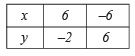Draw the graph using the values of x and y as given in the above table.
At what points the graph of the linear equation cuts the x-axis?

Solution. Plotting the points (6, –2) and (–6, 6) and joining them we get the graph AB which is the required graph.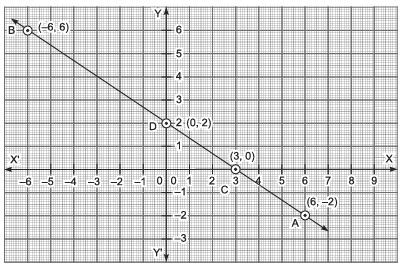The graph AB cuts the x-axis at point C (3, 0) and the y-axis at point D (0, 2).

Question 2. The taxi fare in a town is 10 for the first kilometre and  6 per km for the subsequent distance. Taking the distance as ‘x’ km and total fare as y, write a linear equation for this information, what will be the total fare for 15 km?
Solution:

∵ Total distance is x km.
Total fare = ₹y
∴ x = 1 + (x – 1) = First km + Subsequent distance
Since, fare the first km = ₹10
∴ Fare for the remaining distance = ₹6 x (x – 1) = ₹6x – ₹6
⇒ Total fare = ₹10 + ₹6x – ₹6
= ₹4 + ₹6x
∴ y = 4 + 6x

⇒ y – 6x = 4
⇒ 6x – y + 4 = 0
Which is the required equation.

Now, total fare for 15 km:
6 x 15 – y + 4 = 0   [Substituting x = 15]
⇒ 90 – y + 4 = 0
⇒ 94 – y = 0
⇒ y = 94
∴ Total fare = ₹94.

Question 3. Draw the graph of the equation x – y = 4. From the graph, find the coordinates of the point when the graph line meets the x-axis.
Solution: We have x – y = 4 or y = x – 4
When x = 0, then y = 0 – 4 = –4
When x = 1, then y = 1 – 4 = –3
When x = –1, then y = – 1 – 4 =  – 5
We get the following table of values of x and y.

 x 0 1 -1 y -4 -3 -5 (x, y) (0, –4) (1, –3) (–1, –5)

∴ We have the ordered pairs of solution for x – y = 4 as (0, –4), (1, –3) and (–1, –5).
Now, plotting the points (0, –4), (1, –3) and (–1, –5) and then joining them, we get the following graph of x – y = 4.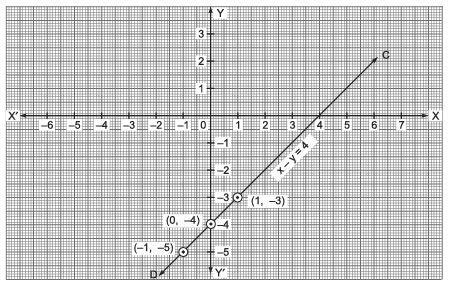From the graph, we find that the graph line meets the x-axis at (4, 0).

Question 4. Draw the graph x + 2y = 6 and from the graph, find the value of x when y = – 3.
Solution: We have: x + 2y = 6
⇒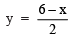When x = 0, then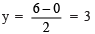When x = 2, then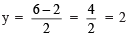When x = 4, then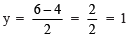We get the following table of values of x and y.

 x 0 2 4 y 3 2 1 (x, y) (0, 3) (2, 2) (4, 1)

Plotting the ordered pairs (0, 3), (2, 2) and (4, 1) and then joining them, we get the graph of x + 2y = 6 as shown below: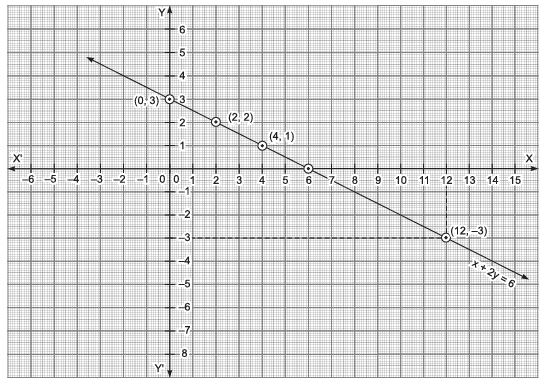From the graph, we find that for y = – 3, the value of x = 12.

The document Long Answer Type Questions: Linear Equations in Two Variables | Mathematics (Maths) Class 9 is a part of the Class 9 Course Mathematics (Maths) Class 9.
All you need of Class 9 at this link: Class 9

## FAQs on Long Answer Type Questions: Linear Equations in Two Variables - Mathematics (Maths) Class 9

 1. What are linear equations in two variables?Ans. Linear equations in two variables are algebraic equations that involve two variables, typically denoted as x and y, and have a degree of 1. These equations can be represented by straight lines on a coordinate plane and have the general form of ax + by = c, where a, b, and c are constants.
 2. How do you solve a system of linear equations in two variables?Ans. To solve a system of linear equations in two variables, you can use one of the following methods: - Substitution method: Solve one equation for one variable and substitute it into the other equation. - Elimination method: Multiply one or both equations by suitable constants to make the coefficients of one variable the same. Then, subtract or add the equations to eliminate one variable. - Graphical method: Plot the equations on a coordinate plane and find the point of intersection, which represents the solution.
 3. What is the importance of linear equations in two variables?Ans. Linear equations in two variables have several applications in real-life situations. Some of the key importance of these equations are: - They help in solving problems involving two unknowns, such as finding the cost and quantity of goods, determining the relationship between two variables, etc. - They provide a mathematical model for various scientific and engineering problems, allowing for prediction and analysis. - They form the basis for learning higher-level concepts in algebra and calculus, making them essential for further mathematical studies.
 4. Can a linear equation in two variables have infinitely many solutions?Ans. Yes, a linear equation in two variables can have infinitely many solutions. This occurs when the two equations represent the same line or are parallel to each other. In such cases, every point on the line is a solution to the system of equations. Graphically, these lines will coincide or never intersect, indicating infinite solutions.
 5. How can linear equations in two variables be used to solve word problems?Ans. Linear equations in two variables can be used to solve various word problems involving real-life scenarios. By assigning variables to unknown quantities and translating the problem into equations, we can solve for the desired values. For example, these equations can be used to find the cost and quantity of items, determine the speed and time of travel, analyze the relationship between two variables, and much more. The solutions obtained from the equations provide a mathematical representation of the problem and aid in making informed decisions.

## Mathematics (Maths) Class 9

62 videos|426 docs|102 tests

## Mathematics (Maths) Class 9

62 videos|426 docs|102 tests
Signup to see your scores go up within 7 days! Learn & Practice with 1000+ FREE Notes, Videos & Tests.
10M+ students study on EduRev
Track your progress, build streaks, highlight & save important lessons and more!(Scan QR code)
Related Searches

,

,

,

,

,

,

,

,

,

,

,

,

,

,

,

,

,

,

,

,

,

;